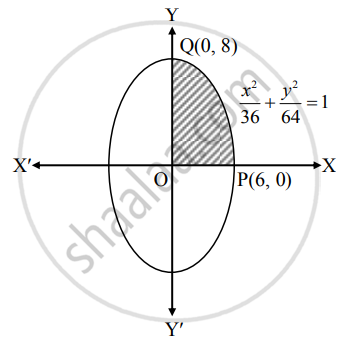# Find the area of the ellipse x236+y264 = 1, using integration - Mathematics and Statistics

Sum

Find the area of the ellipse x^2/36 + y^2/64 = 1, using integration

#### Solution

By the symmetry of the ellipse, required area of the ellipse is 4 times the area of the region OPQO.For the region OPQO, the limits of integration are x = 0 and x = 6.

Given equation of the ellipse is x^2/36 + y^2/64 = 1

∴ y^2/64 = 1 - x^2/36

∴ y2 = 64(1 - x^2/36)

∴ y2 = 64/36(36 - x^2)

∴ y = +-  8/6 sqrt(36 - x^2)

∴ y = 4/3 sqrt(36 - x^2)  ......[∵ In first quadrant, y > 0]

∴ Required area = 4(area of the region OPQO)

= 4int_0^6 y  "d"x

= 4 int_0^6 4/3 sqrt(36 - x^2)  "d"x

= 16/3[x/2 sqrt(36 - x^2) + 36/2 sin^-1 (x/6)]_0^6

= 16/3[6/2 sqrt(36 - 36) + 36/2 sin^-1 (1) - {0 +36/2 sin^-1 (0)}]

= 16/3(0 + 36/2* pi/2 - 0)

= 48π sq.units

Concept: Area Between Two Curves
Is there an error in this question or solution?